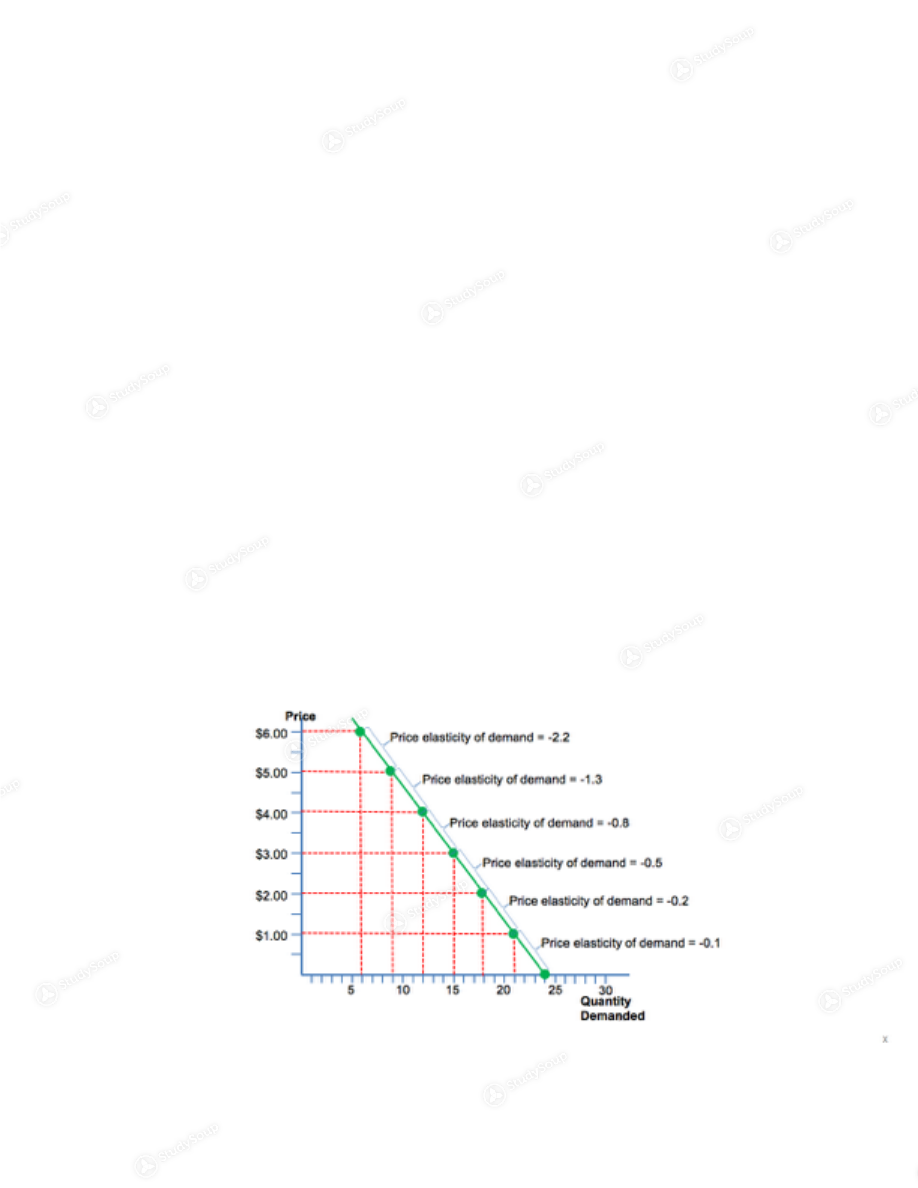Limited time offer 20% OFF StudySoup Subscription details

# MSU - AEC 2713 - Class Notes - Week 5

### Created by: Taylor Baker Elite Notetaker

> > > > MSU - AEC 2713 - Class Notes - Week 5

MSU - AEC 2713 - Class Notes - Week 5

##### Description: this is the beginning of test two material. chapter 5 notes.
This preview shows pages 1 - 4 of a 16 page document. to view the rest of the contentIntro to Food and Resource Economics Week 5: September 12-16, 2016 if you do not understand chapter 4 you need to go back
and review because it is a very important chapter.
Chapter 5: Elasticity and its Application Example:
You own a coffee shop and sell your lattes for the equilibrium
price of \$3 each
However, your input costs are increasing and you are
contemplating a price increase to \$4 per cup
You know that the Law of Demand says that you will sell fewer
cups of coffee if you increase the price, but you don’t know
how many fewer cups you will sell
How much will your revenue fall or increase? Elasticity: Elasticity measures the degree to which one variable (either
price or quantity) responds to changes in the other variable
The Price Elasticity of Demand measures how much the quantity
demanded of lattes will fall if you raise your price
Elasticity is a numerical measure of the responsiveness of
quantity demanded or quantity supplied to one of its
determinants
Price elasticity of demand price elasticity of demand = percentage change in quantity
demanded/ percentage change in price
The Price Elasticity of Demand measures the change in
quantity demanded resulting from a change in price Loosely
speaking, it measures the buyers’ responsiveness to changes
in price
- when you work out a price elasticity of demand problem you will
get a negative number, don't remove the negative because it
illustrates the demand even though the negative is understood.To solve the problem of which way do you move along the curve we
will use a midpoint method to calculate the price elasticity of
demand.
Price elasticity Problem: - Price elasticity of demand = [(Qd2-Qd1)/Qmid]/ [(P2-P1)/Pmid]
- Midpoints
Q mid  = (15 – 12)/2 + 12 = 13.5 P mid  = (\$4 - \$3)/2 + \$3 = \$3.50 - Price Elasticity of demand = [(15-12)/ 13.5]/ [(3-4)/ 3.50] - PE of D = [3/13.5]/ [-1/3.50] - PE of D = 0.22/ -0.29 - PE of D = -0.7 (Keeping in mind the graph below for further understanding)
- The price elasticity of demand changes as you move along the
demand curve
- It is important to realize the portion of the demand curve for
which you are estimating the price elasticity of demand- the negatives show that demand will fall and the number is the
percentage it will fall
Topics regarding the price elasticity of demand: The price elasticity of demand is closely related to the
slope of the demand
The steeper the slope of the demand curve, the smaller
the price elasticity of demand
The flatter the slope of the demand curve, the larger
the price elasticity of demand
Categories of the price elasticity of demand: perfectly inelastic inelastic unitary elastic elastic perfectly elastic In a perfectly inelastic demand (one extreme case): - the demand curve is perfectly vertical - consumer price sensitivity is none - elasticity is 0 - examples include: - pharmaceuticals such as insulin (if you need it you cant
go without it)
- drinking water (you can’t go without it) In a inelastic demand: - If the absolute value of the price elasticity of demand is less
than 1.0, then the demand curve is said to be inelastic
- the demand curve slope is relatively steep - consumer price sensitivity is relatively low- A specific percentage change in price will result in a smaller
percentage change in quantity
- Examples: required textbooks for a college course, gasoline (at
least in the short run) (price doesn’t matter you're still
going to buy it if you want it)
- Price increases (decreases)  - Revenue increases (decreases) - if you have an inelastic demand if you increase price you will
increase revenue. if you decrease price you decrease revenue
.
Unit Elastic Demand: - the demand curve slope is “intermediate” - consumer price sensitivity is “intermediate” - A specific percentage change in price will result in the same
percentage change in quantity
- price change will have no effect on revenue Elastic demand: - If the absolute value of the price elasticity of demand is
greater than 1.0, then the demand curve is said to be elastic
-  the demand curve slope is relatively flat - consumer price sensitivity is relatively high  - a specific percentage change in price will result in a greater
percentage change in quantity
- price increases revenue decreases; price decreases revenue
increases
- Examples:  steak, candy, new automobiles Perfectly elastic demand (the other extreme case) - If the absolute value of the price elasticity of demand is
infinite (∞), then the demand curve is said to be perfectly elastic
-  the demand curve slope is 0 or flat - consumer price sensitivity is relatively high

This is the end of the preview. Please to view the rest of the contentJoin more than 18,000+ college students at Mississippi State University who use StudySoup to get ahead
16 Pages 28 Views 22 Unlocks
• Notes, Study Guides, Flashcards + More!Join more than 18,000+ college students at Mississippi State University who use StudySoup to get ahead
##### Description: this is the beginning of test two material. chapter 5 notes.
16 Pages 28 Views 22 Unlocks
• Notes, Study Guides, Flashcards + More!
Get Full Access to MSU - AEC 2713 - Class Notes - Week 5
×
Get Full Access to MSU - AEC 2713 - Class Notes - Week 5

I don't want to reset my password

Need help? Contact support

Need an Account? Is not associated with an account
We're here to help